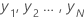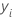# Methods and formulas for the response information in Fit Model and Discover Key Predictors with TreeNet® Regression

###### Note

The values in the response information table are for the response variable and do not depend on the validation method. The statistics take weights into account.

## N

The number of non-missing values.

## % of N

Percentage of the total number of observations in the training data set and the test data set.

## Mean

A commonly used measure of the center of a batch of numbers. The mean is also called the average. It is the sum of all observations divided by the number of (nonmissing) observations.

## StDev

If the data set containswith mean, then the standard deviation of the sample is:
TermDescriptionobservationmean of the observations
N number of nonmissing observations

## Minimum

The smallest value in the data set.

## Q1

25% of your sample observations are less than or equal to the value of the 1st quartile. Therefore, the 1st quartile is also referred to as the 25th percentile.

## Median

The sample median is in the middle of the data: at least half the observations are less than or equal to it, and at least half are greater than or equal to it.

Suppose you have a data set that contains N values. To calculate the median, first order your data values from smallest to largest. If N is odd, the sample median is the value in the middle. If N is even, the sample median is the average of the two middle values.

## Q3

75% of your sample observations are less than or equal to the value of the third quartile. Therefore, the third quartile is also referred to as the 75th percentile.

## Maximum

The largest value in the data set.